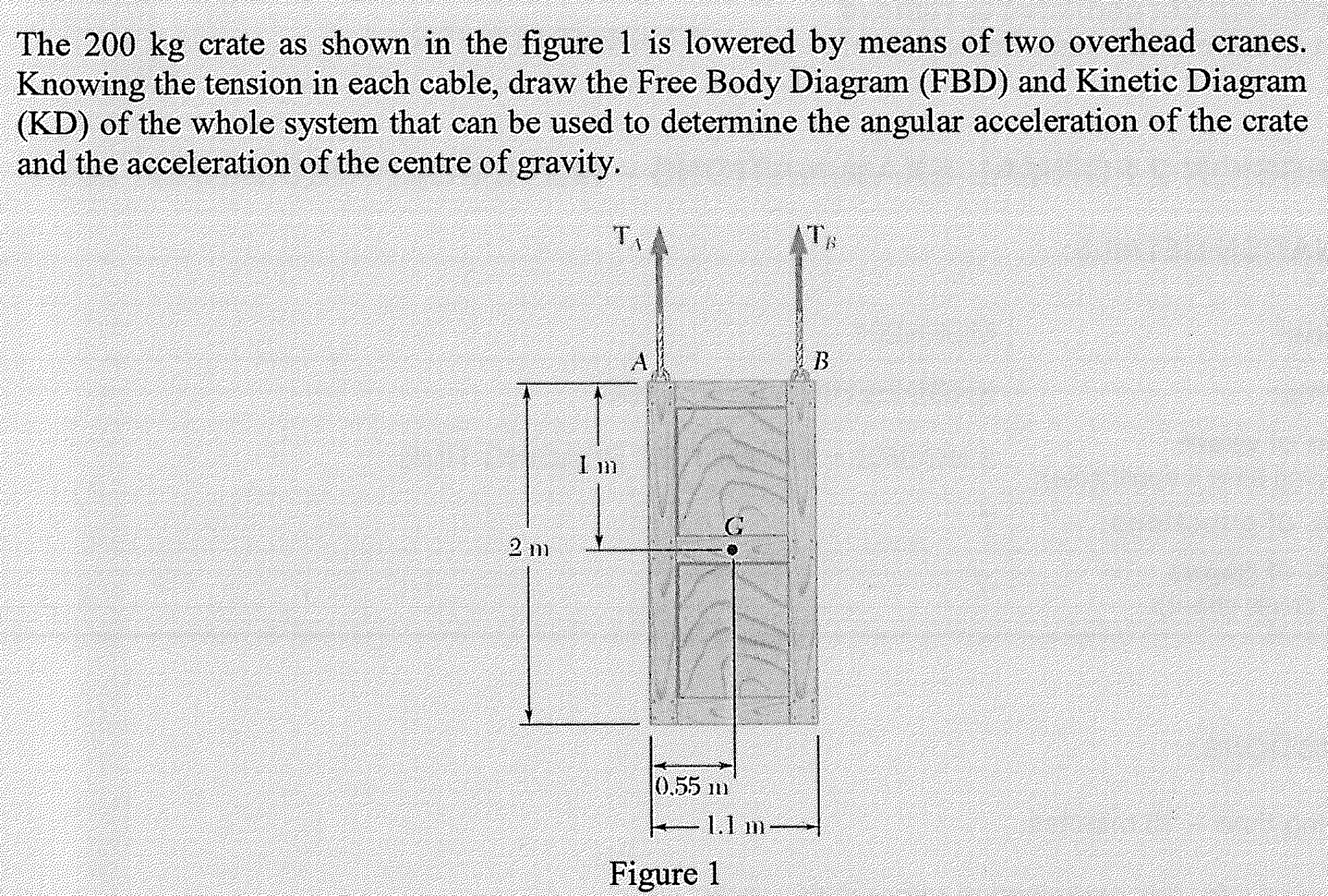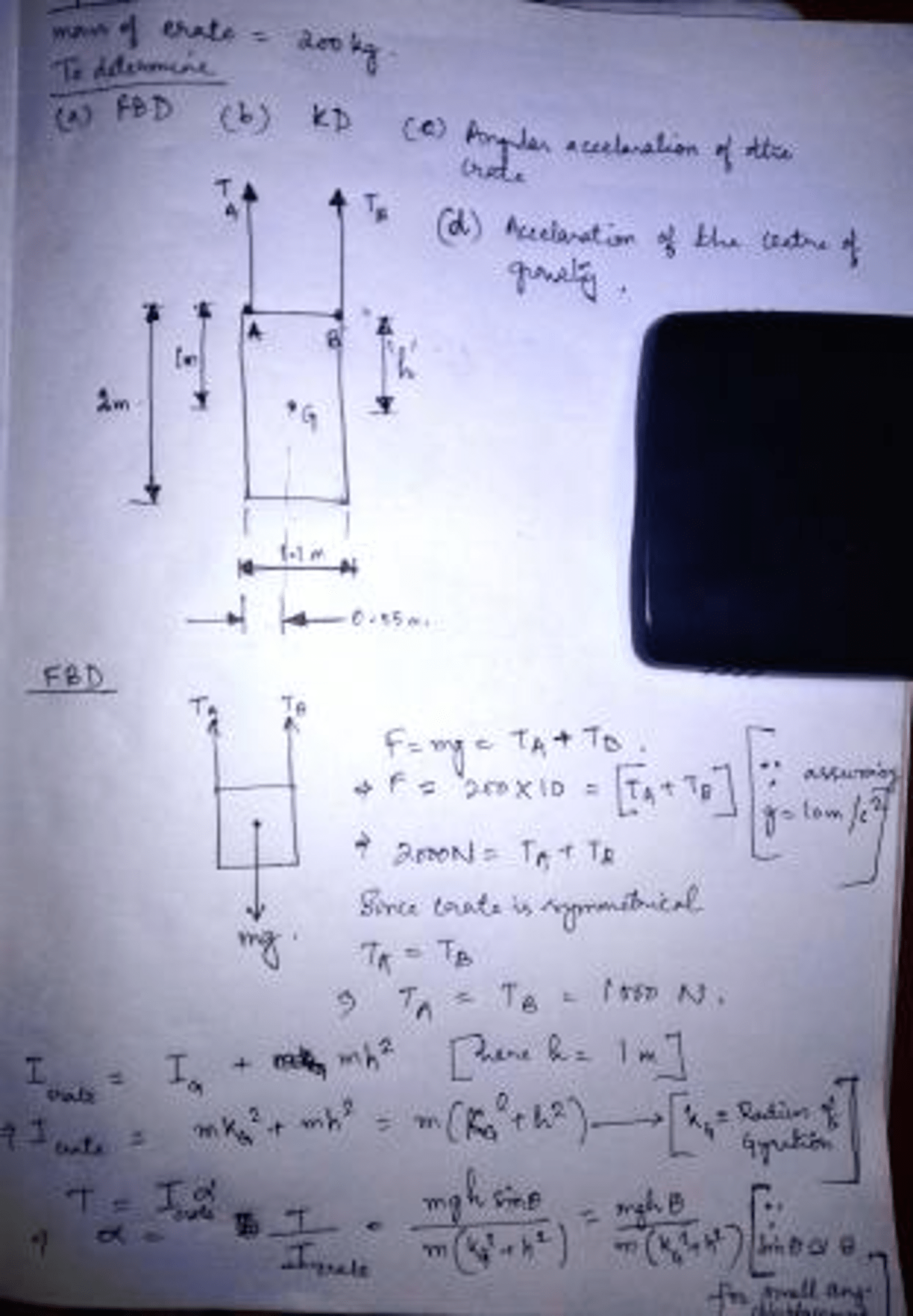# The 200 kg crate as shown in the figure 1 is lowered by means of two overhead cranes. Knowing the tension in each cable, draw the Free Body Diagram (FBD) and Kinetic Diagram (KD) of the whole system that can be used to determine the angular acceleration of the crate and the acceleration of the centre of gravity.

Question-AnswerCategory: Engineering MechanicsThe 200 kg crate as shown in the figure 1 is lowered by means of two overhead cranes. Knowing the tension in each cable, draw the Free Body Diagram (FBD) and Kinetic Diagram (KD) of the whole system that can be used to determine the angular acceleration of the crate and the acceleration of the centre of gravity.

The 200 kg crate as shown in the figure 1 is lowered by means of two overhead cranes. Knowing the tension in each cable, draw the Free Body Diagram (FBD) and Kinetic Diagram (KD) of the whole system that can be used to determine the angular acceleration of the crate and the acceleration of the centre of gravity.